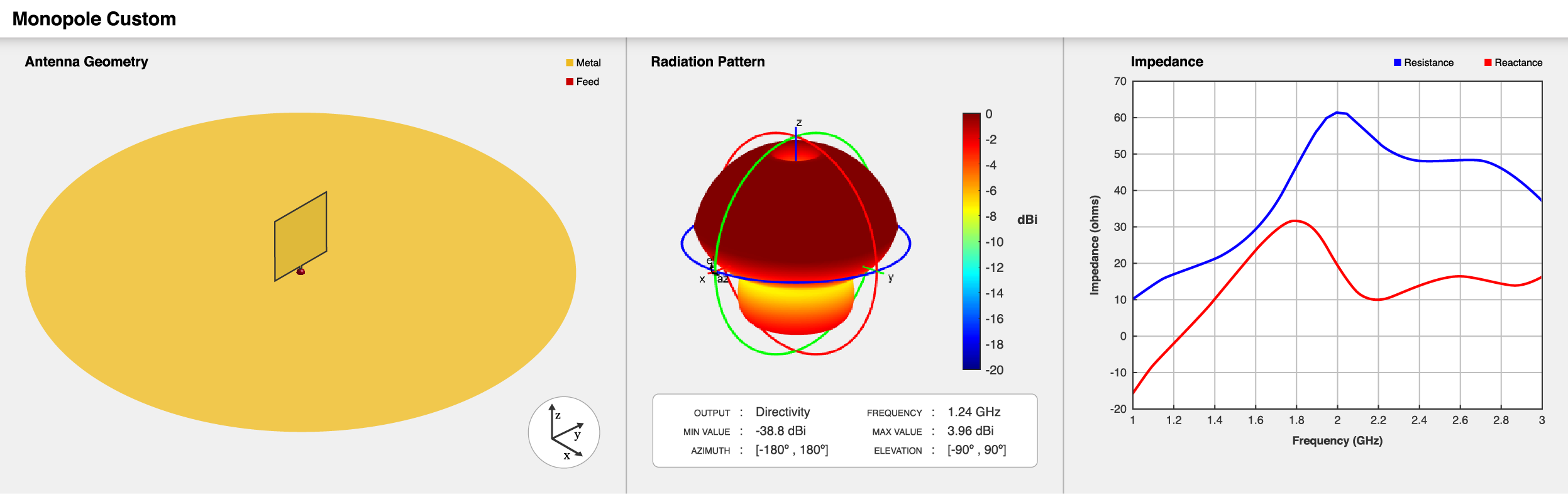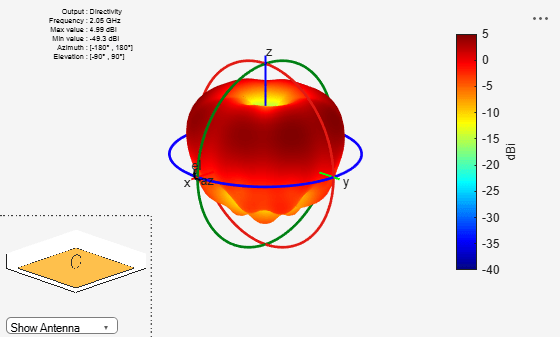# monopoleCustom

Create customized monopole antenna

Since R2020b

## Description

The `monopoleCustom` object creates a monopole radiator of any shape using the `antenna.Shape` class. The ground plane can take any shape. You can create any arbitrarily shaped monopole and analyze it for field, surface, and port characteristics. Monopole antennas have a simple structure and provide omnidirectional radiation patterns with wide impedance bandwidth. Monopole antennas are commonly used in airborne and ground-based communication systems.## Creation

### Syntax

``ant = monopoleCustom``
``ant = monopoleCustom(Name,Value)``

### Description

example

````ant = monopoleCustom` creates a default monopole antenna with a square radiator and a circular ground plane. The feed point is at the origin in the xy- plane. The default antenna resonates at an operating frequency of 1.24 GHz.```
````ant = monopoleCustom(Name,Value)` sets Properties using one or more name-value pairs. For example, ```ant = monopoleCustom('RadiatorTilt',90)``` creates a monopole antenna with tilt angle of the radiator at 90 degrees on the z-axis.```

## Properties

expand all

Type of radiator, specified as an `antenna.Polygon` object. You can specify any shape for the radiator. The feed strip is a part of the radiator. By default, the radiator is square in shape with a side length of 40e-3 meters. The feed strip is 2e-3 meters in length and 2.5e-3 meters in width at the edge of the radiator.

Type of ground plane, specified as an `antenna.Polygon` object. You can specify any shape for the ground plane. By default, the ground plane is circular in shape with a radius of 150e-3 meters.

Signed distance from the center along the length and the width of the ground plane, specified as a two-element vector in meters.

Example: `'FeedOffset',[2 1]`

Data Types: `double`

Tilt angle of the radiator, specified as a scalar in degrees.

Data Types: `double`

Type of the metal used as a conductor, specified as a metal material object. You can choose any metal from the `MetalCatalog` or specify a metal of your choice. For more information, see `metal`. For more information on metal conductor meshing, see Meshing.

Example: `m = metal('Copper'); 'Conductor',m`

Example: `m = metal('Copper'); ant.Conductor = m`

Tilt angle of the antenna, specified as a scalar or vector with each element unit in degrees. For more information, see Rotate Antennas and Arrays.

Example: `'Tilt',90`

Example: `ant.Tilt = 90`

Example: `'Tilt',[90 90]`,```'TiltAxis',[0 1 0;0 1 1]``` tilts the antenna at 90 degrees about the two axes defined by the vectors.

Data Types: `double`

Tilt axis of the antenna, specified as:

• Three-element vector of Cartesian coordinates in meters. In this case, each coordinate in the vector starts at the origin and lies along the specified points on the x-, y-, and z-axes.

• Two points in space, each specified as three-element vectors of Cartesian coordinates. In this case, the antenna rotates around the line joining the two points in space.

• A string input describing simple rotations around one of the principal axes, 'x', 'y', or 'z'.

Example: `'TiltAxis',[0 1 0]`

Example: `'TiltAxis',[0 0 0;0 1 0]`

Example: `ant.TiltAxis = 'Z'`

Lumped elements added to the antenna feed, specified as a `lumpedElement` object. You can add a load anywhere on the surface of the antenna. By default, the load is at the feed. For more information, see `lumpedElement`.

Example: `'Load',lumpedElement`. `lumpedElement` is the object for the load created using `lumpedElement`.

Example: `ant.Load = lumpedElement('Impedance',75)`

## Object Functions

 `show` Display antenna, array structures or shapes `axialRatio` Axial ratio of antenna `beamwidth` Beamwidth of antenna `charge` Charge distribution on antenna or array surface `current` Current distribution on antenna or array surface `efficiency` Radiation efficiency of antenna `EHfields` Electric and magnetic fields of antennas; Embedded electric and magnetic fields of antenna element in arrays `impedance` Input impedance of antenna; scan impedance of array `mesh` Mesh properties of metal, dielectric antenna, or array structure `optimize` Optimize antenna or array using SADEA optimizer `pattern` Display antenna radiation pattern in Site Viewer `patternAzimuth` Azimuth pattern of antenna or array `patternElevation` Elevation pattern of antenna or array `rcs` Calculate and plot radar cross section (RCS) of platform, antenna, or array `returnLoss` Return loss of antenna; scan return loss of array `sparameters` Calculate S-parameter for antenna and antenna array objects `vswr` Voltage standing wave ratio of antenna

## Examples

collapse all

Create a disc monopole with a of radius 25 mm, on a square ground plane of 30 cm, and with a feed gap of 0.7 mm.

``` Rad = antenna.Circle('Radius',25e-3); FeedStrip = antenna.Rectangle('Length',1e-3,'Width',0.7e-3, ... 'Center',[0 -(Rad.Radius+(0.7e-3)*0.3)]); m = monopoleCustom; m.Radiator = Rad+FeedStrip; m.GroundPlane = antenna.Rectangle('Length',300e-3,'Width',300e-3); ```

View the antenna using the `show` function.

` show(m); `Plot the radiation pattern of the antenna at 2.05 GHz.

```p = PatternPlotOptions('MagnitudeScale',[-40 5]); pattern(m,2.05e9,'patternOptions',p);```Ammann, M. J. “Square Planar Monopole Antenna.” IEE National Conference on Antennas and Propagation, vol. 1999, IEE, pp. 37–40.

 Weiner, M. “Monopole Element at the Center of a Circular Ground Plane Whose Radius Is Small or Comparable to a Wavelength.” IEEE Transactions on Antennas and Propagation, vol. 35, no. 5, pp. 488–495.

 N. P. Agrawall, G. Kumar and K. P. Ray, "Wide-band planar monopole antennas," in IEEE Transactions on Antennas and Propagation, vol. 46, no. 2, pp. 294-295.